# 导师计划--数据结构和算法系列（上）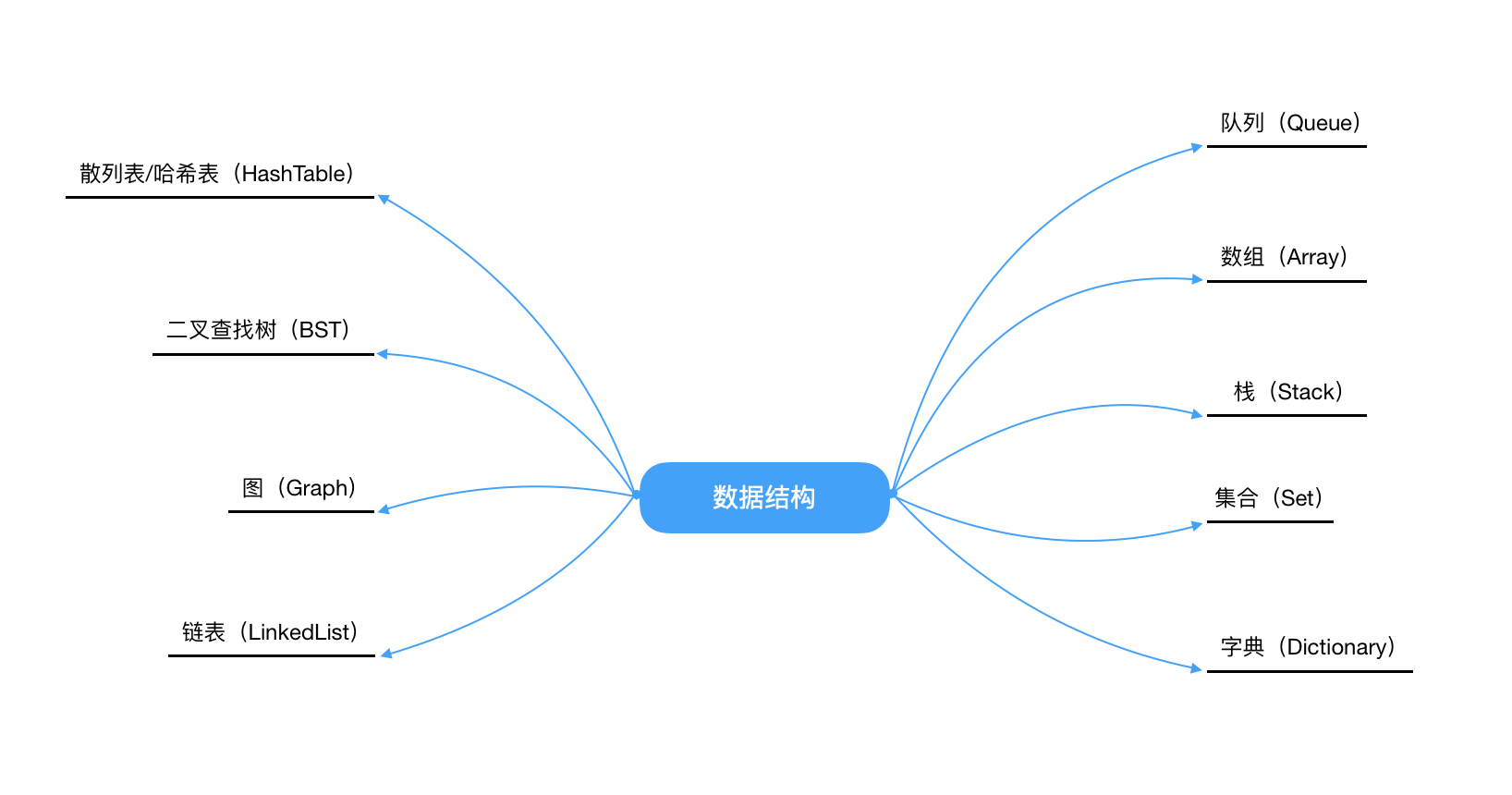1.线性结构是一个有序数据元素的集合。它应该满足下面的特征：

• 集合中必存在唯一的一个“第一个元素”
• 集合中必存在唯一的一个“最后的元素”
• 除最后一元素之外，其它数据元素均有唯一的“后继”
• 除第一个元素之外，其它数据元素均有唯一的“前驱”

2.非线性结构其逻辑特征是一个节点元素可以有多个直接前驱或多个直接后继。

### 数组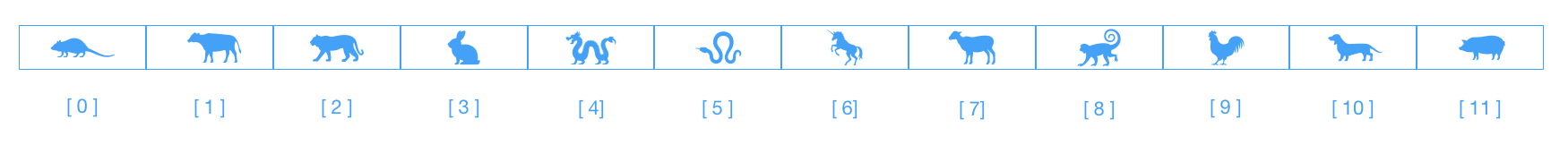``````javascript
let arr = ['鼠', '牛', '虎', '兔', '龙', '蛇', '马', '羊', '猴', '鸡', '狗', '猪'];
arr.forEach((item, index) => {
console.log(`[ \${index} ] => \${item}`);
});

// [ 0 ] => 鼠
// [ 1 ] => 牛
// [ 2 ] => 虎
// [ 3 ] => 兔
// [ 4 ] => 龙
// [ 5 ] => 蛇
// [ 6 ] => 马
// [ 7 ] => 羊
// [ 8 ] => 猴
// [ 9 ] => 鸡
// [ 10 ] => 狗
// [ 11 ] => 猪
``````

• length : 表示数组的长度

• splice(index, howmany, item, ... itemx)

splice方法自认为是数组中最强大的方法。可以实现数组元素的添加、删除和替换。参数`index`为整数且必需，规定添加/删除项目的位置，使用负数可从数组结尾处规定位置；参数`howmany`为必需，为要删除的项目数量，如果设置为 0，则不会删除项目；`item1, ... itemx`为可选，向数组添加新的项目。

• indexOf(searchValue, fromIndex)

indexOf方法返回某个指定字符串值在数组中的位置。`searchValue`是查询的字符串；`fromIndex`是查询的开始位置，默认是0。如果查询不到，会返回-1。

• concat(array1, ... arrayn)

concat方法用于连接两个或者多个数组。

• push(newElement1, ... newElementN)

push方法可向`数组的末尾`添加一个或者多个元素。

• unshift(newElement1, ... newElementN)

unshift方法可向`数组的开头`添加一个或者多个元素。

• pop()

pop方法用于删除并返回`数组的最后一个元素`

• shift()

shift方法可以删除数组的`第一个元素`

• reverse()

reverse方法用于数组的反转

• sort(sortFn)

sort方法是对数组的元素排序。参数`sortFn`可选，其规定排序顺序，必须是函数。

``````javascript
let values = [0, 1, 5, 10, 15];
values.sort();
console.log(values); // [0, 1, 10, 15, 5]
// 为什么会出现这种排序结果呢❓
// 因为在忽略sortFn的情况下，元素会按照转换为字符串的各个字符的Unicode位点进行排序，如下
let equalValues = ['0', '1', '5', '10', '15'];
equalValues.sort();
console.log(equalValues); //  ["0", "1", "10", "15", "5"]

let arr = [0, 10, 5, 1, 15];
function compare(el1, el2){
return el1 - el2; // 升序排列
}
arr.sort(compare);
console.log(arr); // [0, 1, 5, 10, 15]

arr.sort((el1, el2) => {
return el2 - el1; // 降序排列
});
console.log(arr); // [15, 10, 5, 1, 0]
``````
• forEach(fn(currentValue, index, arr), thisValue)

forEach方法用于调用数组的每个元素，并将元素传递给回调函数。参数`function(currentValue, index, arr){}`是一个回调函数。`thisValue`可选，传递给函数的值一般用 "this" 值，如果这个参数为空， "undefined" 会传递给 "this" 值。

• every(fn(currentValue, index, arr), thisValue)

every方法用于检测数组中所有元素是否符合指定条件，如果数组中检测到有一个元素不满足，则整个表达式返回`false`，且剩余的元素不再检查。如果所有的元素都满足条件，则返回`true`

• some(fn(currentValue,index,arr),thisValue)

some方法用于检测数组中元素是否满足指定条件。只要有一个符合就返回`true`，剩余的元素不再检查。如果所有元素都不符合条件，则返回`false`

• reduce(fn(accumulator, currentValue, currentIndex, arr), initialValue)

reduce方法接收一个函数作为累加器，数组中的每个值（从左到右）开始缩减，最终为一个值。回调函数的四个参数的意义如下：`accumulator`，必需，累计器累计回调的返回值, 它是上一次调用回调时返回的累积值，或initialValue；`currentValue`，必需，数组中正在处理的元素；`currentIndex`,可选,数组中正在处理的当前元素的索引，如果提供了initialValue，则起始索引号为0，否则为1;`arr`,可选,当前元素所属的数组对象。`initialValue`，可选，传递给函数的初始值。

``````javascript
let arr = [1, 2, 3, 4];
let reducer = (accumulator, currentValue) => accumulator + currentValue;

// 1 + 2 + 3 + 4
console.log(arr.reduce(reducer)); // 10

// 5 + 1 + 2 + 3 + 4
console.log(arr.reduce(reducer, 5)); // 15
``````

### 栈

• LIFO(Last In First Out)表示后进先出，后进来的元素第一个弹出栈空间。类似于自动餐托盘，最后放上去的托盘，往往先被拿出来使用。
• 仅允许在表的一端进行插入和移除元素。这一端被称为栈顶，相对地，把另一端称为栈底。如下图的标识。
• 向一个栈插入新元素称作进栈、入栈或压栈，这是将新元素放在栈顶元素上面，使之成为新的栈顶元素。
• 从一个栈删除元素又称为出栈或退栈，它是把栈顶元素删除掉，使其相邻的元素成为新的栈顶元素。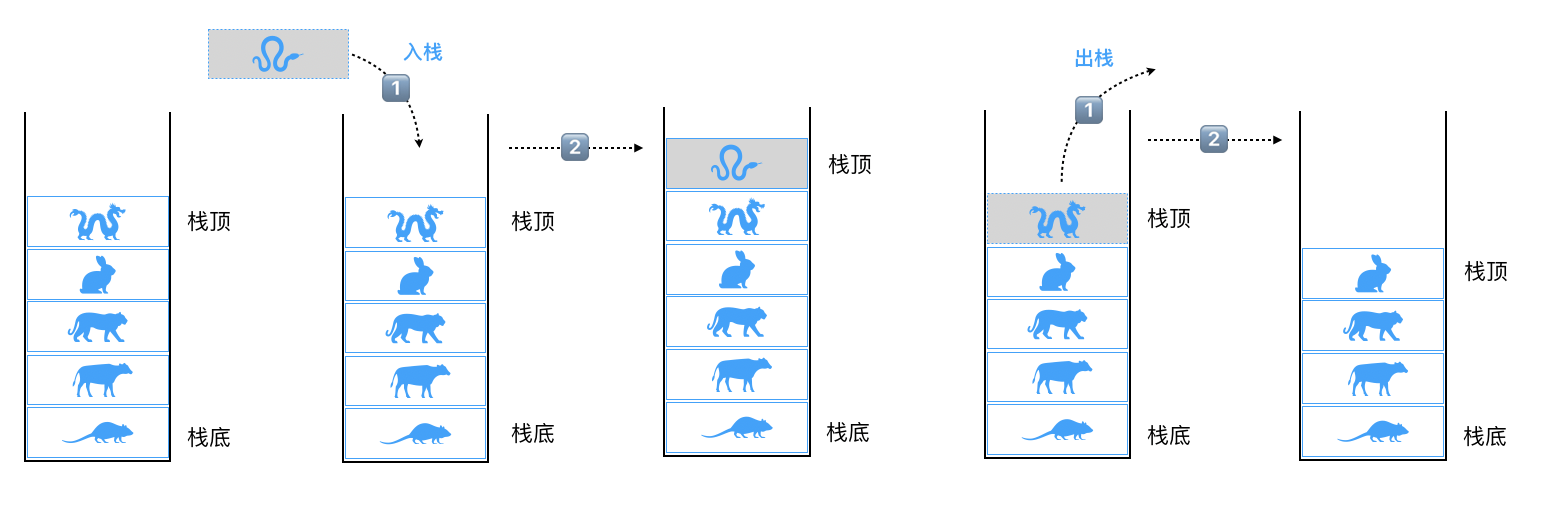``````javascript
class Stack {
constructor(){
this.items = [];
}
// 入栈操作
push(element = ''){
if(!element) return;
this.items.push(element);
return this;
}
// 出栈操作
pop(){
this.items.pop();
return this;
}
// 对栈一瞥，理论上只能看到栈顶或者说即将处理的元素
peek(){
return this.items[this.size() - 1];
}
// 打印栈数据
print(){
return this.items.join(' ');
}
// 栈是否为空
isEmpty(){
return this.items.length == 0;
}
// 返回栈的元素个数
size(){
return this.items.length;
}
}
let stack = new Stack(),
arr = ['鼠', '牛', '虎', '兔', '龙', '蛇', '马', '羊', '猴', '鸡', '狗', '猪'];
arr.forEach(item => {
stack.push(item);
});
console.log(stack.print()); // 鼠 牛 虎 兔 龙 蛇 马 羊 猴 鸡 狗 猪
console.log(stack.peek()); // 猪
stack.pop().pop().pop().pop();
console.log(stack.print()); // 鼠 牛 虎 兔 龙 蛇 马 羊
console.log(stack.isEmpty()); // false
console.log(stack.size()); // 8
``````

⚠️ 注意：栈这里的push和pop方法要和数组方法的push和pop方法区分下。

### 队列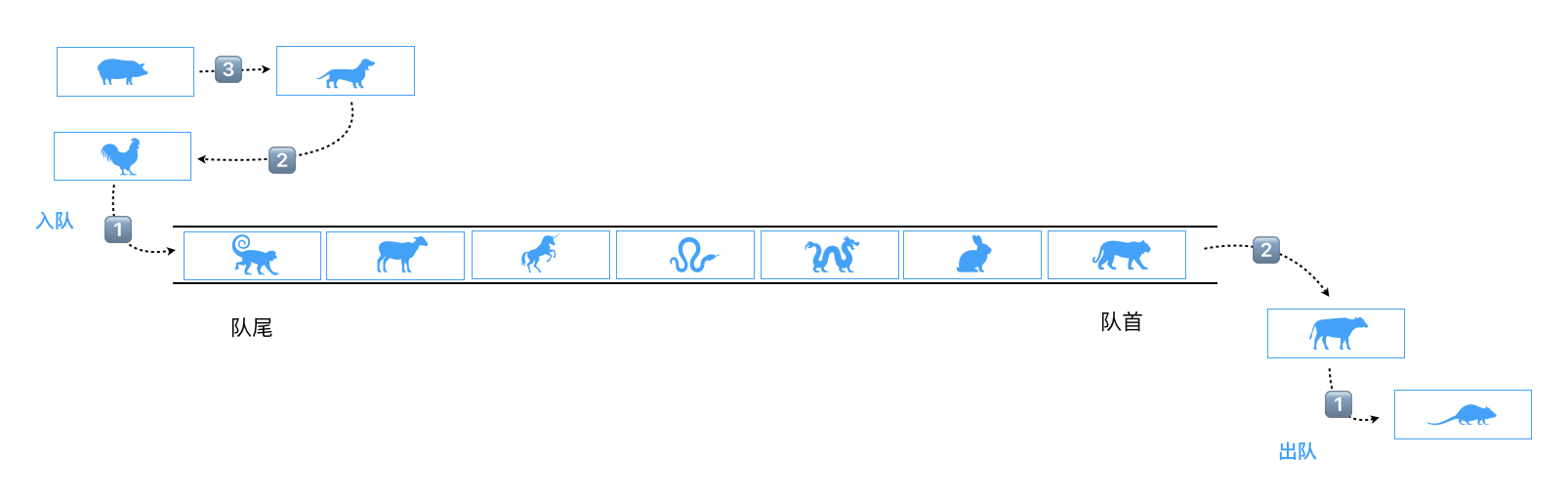``````javascript
class Queue {
constructor(){
this.items = [];
}
// 入队操作
enqueue(element = ''){
if(!element) return;
this.items.push(element);
return this;
}
// 出队操作
dequeue(){
this.items.shift();
return this;
}
// 查看队前元素或者说即将处理的元素
front(){
return this.items;
}
// 查看队列是否为空
isEmpty(){
return this.items.length == 0;
}
// 查看队列的长度
len(){
return this.items.length;
}
// 打印队列数据
print(){
return this.items.join(' ');
}
}

let queue = new Queue(),
arr = ['鼠', '牛', '虎', '兔', '龙', '蛇', '马', '羊', '猴', '鸡', '狗', '猪'];
arr.forEach(item => {
queue.enqueue(item);
});
console.log(queue.print()); // 鼠 牛 虎 兔 龙 蛇 马 羊 猴 鸡 狗 猪
console.log(queue.isEmpty()); // false
console.log(queue.len()); // 12
queue.dequeue().dequeue();
console.log(queue.front()); // 虎
console.log(queue.print()); // 虎 兔 龙 蛇 马 羊 猴 鸡 狗 猪
``````

### 链表

• 存储多个元素，比较常用
• 访问便捷，使用下标[index]即可访问

• 数组的创建通常需要申请一段连续的内存空间，并且大小是固定的（大多数的编程语言数组都是固定的），所以在进行扩容的时候难以掌控。（一般情况下，申请一个更大的数组，会是之前数组的倍数，比如两倍。然后，再将原数组中的元素复制过去）
• 插入数据越是靠前，其成本很高，因为需要进行大量元素的位移。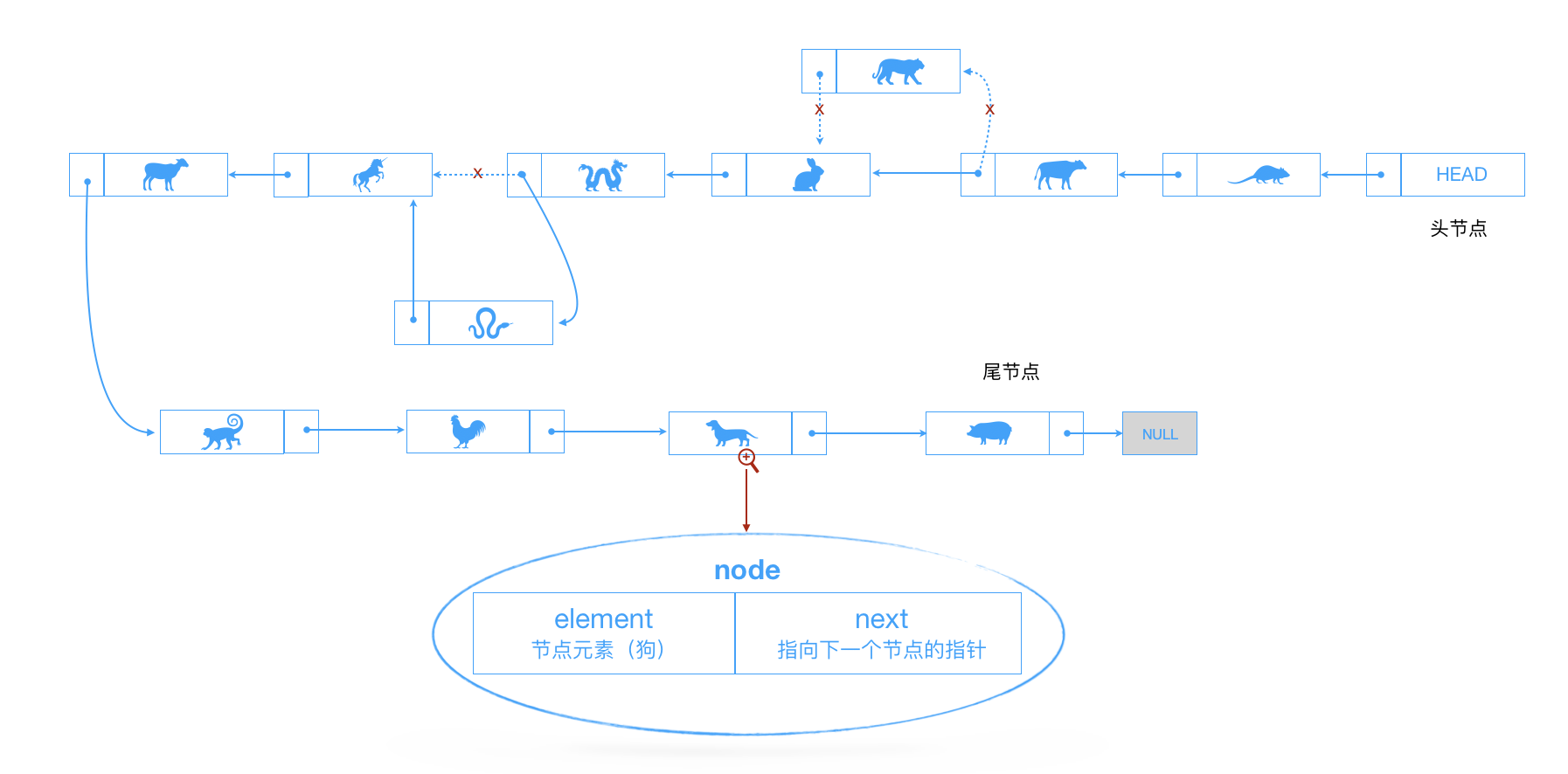``````javascript
// 链表
class Node {
constructor(element){
this.element = element;
this.next = null;
}
}

class LinkedList {
constructor(){
this.length = 0; // 链表长度
this.head = new Node('head'); // 表头节点
}
/**
*  find 查找元素的功能，找不到的情况下直接返回链尾节点
*  { String } item 要查找的元素
*  { Object } 返回查找到的节点
*/
find(item = ''){
let currNode = this.head;
while(currNode.element != item && currNode.next){
currNode = currNode.next;
}
return currNode;
}
/**
*  findPrevious 查找链表指定元素的前一个节点
*  { String } item 指定的元素
*  { Object } 返回查找到的之前元素的前一个节点，找不到节点的话返回链尾节点
*/
findPrevious(item){
let currNode = this.head;
while((currNode.next != null) && (currNode.next.element != item)){
currNode = currNode.next;
}
return currNode;
}
/**
*  insert 插入功能
*  { String } newElement 要出入的元素
*  { String } item 想要追加在后的元素（此元素不一定存在）
*/
insert(newElement = '', item){
if(!newElement) return;
let newNode = new Node(newElement),
currNode = this.find(item);
newNode.next = currNode.next;
currNode.next = newNode;
this.length++;
return this;
}
// 展示链表元素
display(){
let currNode = this.head,
arr = [];
while(currNode.next != null){
arr.push(currNode.next.element);
currNode = currNode.next;
}
return arr.join(' ');
}
// 链表的长度
size(){
return this.length;
}
// 查看链表是否为空
isEmpty(){
return this.length == 0;
}
/**
*  indexOf 查看链表中元素的索引
*  { String } element 要查找的元素
*/
indexOf(element){
let currNode = this.head,
index = 0;
while(currNode.next != null){
index++;
if(currNode.next.element == element){
return index;
}
currNode = currNode.next;
}
return -1;
}
/**
*  removeEl 移除指定的元素
*  { String } element
*/
removeEl(element){
let preNode = this.findPrevious(element);
preNode.next = preNode.next != null ? preNode.next.next : null;
this.length--;
}
}

let linkedlist = new LinkedList();
console.log(linkedlist.isEmpty()); // true
linkedlist.insert('鼠').insert('虎').insert('牛', '鼠');
console.log(linkedlist.display()); // 鼠 牛 虎
console.log(linkedlist.find('猪')); // Node { element: '虎', next: null }
console.log(linkedlist.find('鼠')); // Node { element: '鼠', next: Node { element: '牛', next: Node { element: '虎', next: null } } }
console.log(linkedlist.size()); // 3
console.log(linkedlist.indexOf('鼠')); // 1
console.log(linkedlist.indexOf('猪')); // -1
console.log(linkedlist.findPrevious('虎')); // Node { element: '牛', next: Node { element: '虎', next: null } }
linkedlist.removeEl('鼠');
console.log(linkedlist.display()); // 牛 虎
``````

### 字典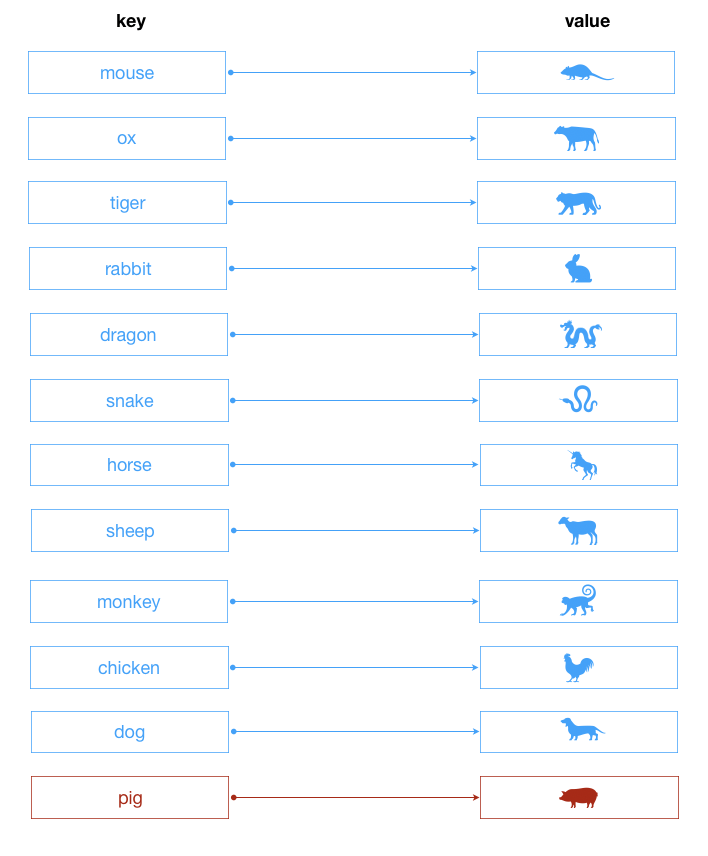``````javascript
class Dictionary {
constructor(){
this.items = {};
}
/**
*  set 设置字典的键值对
*  { String } key 键
*  {*} value 值
*/
set(key = '', value = ''){
this.items[key] = value;
return this;
}
/**
*  get 获取某个值
*  { String } key 键
*/
get(key = ''){
return this.has(key) ? this.items[key] : undefined;
}
/**
*  has 判断是否含有某个键的值
*  { String } key 键
*/
has(key = ''){
return this.items.hasOwnProperty(key);
}
/**
*  remove 移除元素
*  { String } key
*/
remove(key){
if(!this.has(key))  return false;
delete this.items[key];
return true;
}
// 展示字典的键
keys(){
return Object.keys(this.items).join(' ');
}
// 字典的大小
size(){
return Object.keys(this.items).length;
}
// 展示字典的值
values(){
return Object.values(this.items).join(' ');
}
// 清空字典
clear(){
this.items = {};
return this;
}
}

let dictionary = new Dictionary(),
// 这里需要修改
arr = [{ key: 'mouse', value: '鼠'}, {key: 'ox', value: '牛'}, {key: 'tiger', value: '虎'}, {key: 'rabbit', value: '兔'}, {key: 'dragon', value: '龙'}, {key: 'snake', value: '蛇'}, {key: 'horse', value: '马'}, {key: 'sheep', value: '羊'}, {key: 'monkey', value: '猴'}, {key: 'chicken', value: '鸡'}, {key: 'dog', value: '狗'}, {key: 'pig', value: '猪'}];
arr.forEach(item => {
dictionary.set(item.key, item.value);
});
console.log(dictionary.keys()); // mouse ox tiger rabbit dragon snake horse sheep monkey chicken dog pig
console.log(dictionary.values()); // 鼠 牛 虎 兔 龙 蛇 马 羊 猴 鸡 狗 猪
console.log(dictionary.has('dragon')); // true
console.log(dictionary.get('tiger')); // 虎
console.log(dictionary.remove('pig')); // true
console.log(dictionary.size()); // 11
console.log(dictionary.clear().size()); // 0
``````

### 集合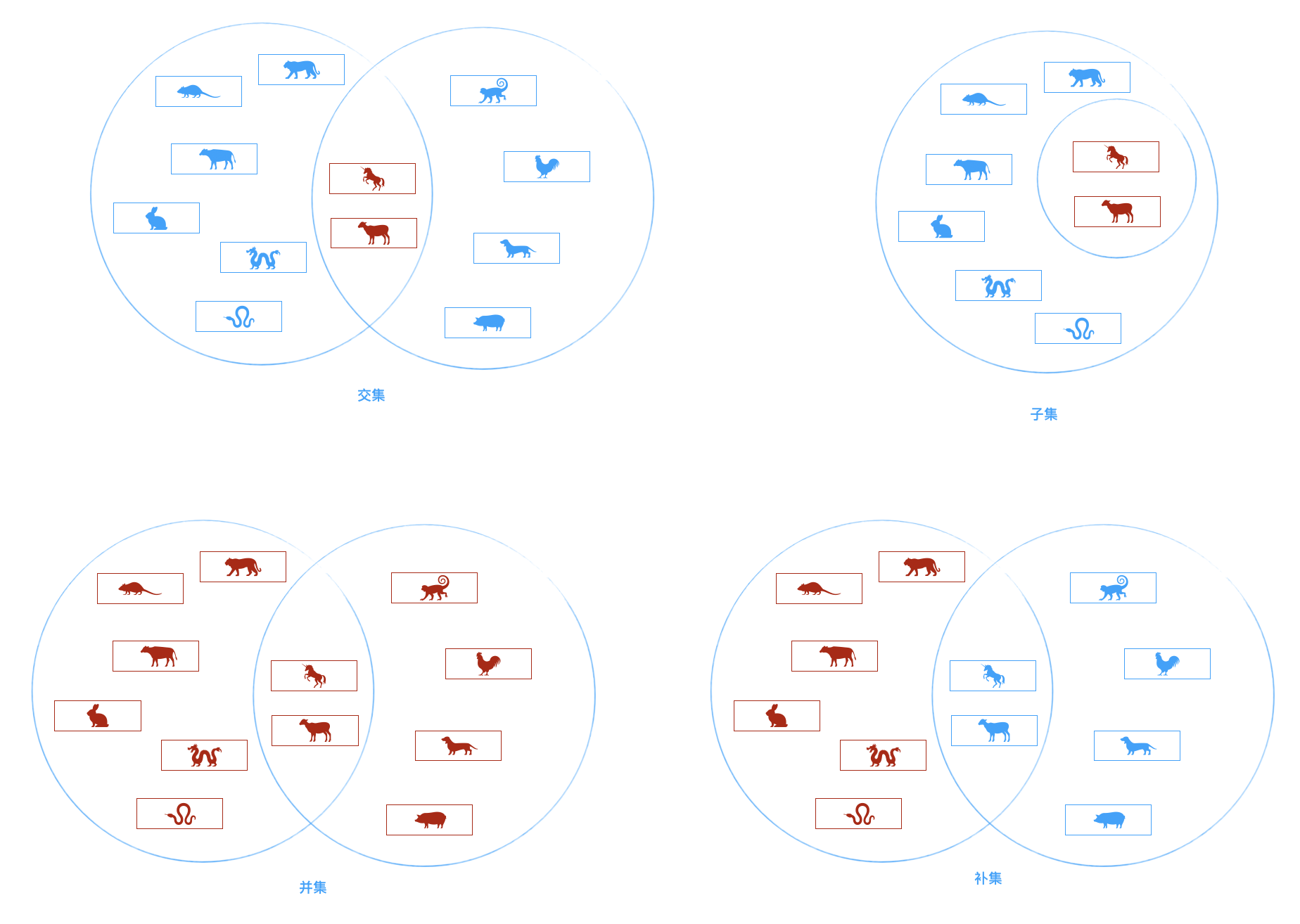es6中已经封装好了可用的Set类。我们手动来写下相关的逻辑：

``````javascript
// 集合
class Set {
constructor(){
this.items = [];
}
/**
*  add 添加元素
*  { String } element
*  { Boolean }
*/
add(element = ''){
if(this.items.indexOf(element) >= 0) return false;
this.items.push(element);
return true;
}
// 集合的大小
size(){
return this.items.length;
}
// 集合是否包含某指定元素
has(element = ''){
return this.items.indexOf(element) >= 0;
}
// 展示集合
show(){
return this.items.join(' ');
}
// 移除某个元素
remove(element){
let pos = this.items.indexOf(element);
if(pos < 0) return false;
this.items.splice(pos, 1);
return true;
}
/**
*  union 并集
*  { Array } set 数组集合
*  { Object } 返回并集的对象
*/
union(set = []){
let tempSet = new Set();
for(let i = 0; i < this.items.length; i++){
tempSet.add(this.items[i]);
}
for(let i = 0; i < set.items.length; i++){
if(tempSet.has(set.items[i])) continue;
tempSet.items.push(set.items[i]);
}
return tempSet;
}
/**
*  intersect 交集
*  { Array } set 数组集合
*  { Object } 返回交集的对象
*/
intersect(set = []){
let tempSet = new Set();
for(let i = 0; i < this.items.length; i++){
if(set.has(this.items[i])){
tempSet.add(this.items[i]);
}
}
return tempSet;
}
/**
*  isSubsetOf 【A】是【B】的子集❓
*  { Array } set 数组集合
*  { Boolean } 返回真假值
*/
isSubsetOf(set = []){
if(this.size() > set.size()) return false;
this.items.forEach*(item => {
if(!set.has(item)) return false;
});
return true;
}
}

let set = new Set(),
arr = ['鼠', '牛', '虎', '兔', '龙', '蛇', '马', '羊', '猴'];
arr.forEach(item => {
set.add(item);
});
console.log(set.show()); // 鼠 牛 虎 兔 龙 蛇 马 羊 猴
console.log(set.has('猪')); // false
console.log(set.size()); // 9
set.remove('鼠');
console.log(set.show()); // 牛 虎 兔 龙 蛇 马 羊 猴
let setAnother = new Set(),
anotherArr = ['马', '羊', '猴', '鸡', '狗', '猪'];
anotherArr.forEach(item => {
setAnother.add(item);
});
console.log(set.union(setAnother).show()); // 牛 虎 兔 龙 蛇 马 羊 猴 鸡 狗 猪
console.log(set.intersect(setAnother).show()); // 马 羊 猴
console.log(set.isSubsetOf(setAnother)); // false
``````

### 散列表/哈希表

• 方案一：数组

• 按照顺序将所有员工信息依次存入一个长度为1000的数组中。每个员工的信息都保存在该数组的某个位置上。
• 但是我们要查看某个员工的信息怎么办呢？一个个查找吗？不太好找。
• 数组最大的优势是什么？通过下标值获取信息。
• 所以为了可以通过数组快速定位到某个员工，最好给员工信息中添加一个员工编号，而`编号`对应的就是员工的`下标值`
• 当查找某个员工信息时，通过员工号可以快速定位到员工的信息位置。
• 方案二：链表

• 链表对应插入和删除数据有一定的优势。
• 但是对于获取员工的信息，每次都必须从头遍历到尾，这种方式显然不是特别适合我们这里。
• 最终方案：

• 这么看最终方案似乎就是数组了，但是数组还是有缺点，什么缺点呢？
• 假如我们想查看下张三这位员工的信息，但是我们不知道张三的员工编号，怎么办呢？
• 当然，我们可以问他的员工编号。但是我们每查找一个员工都是要问一下这个员工的编号吗？不合适。【那我们还不如直接问他的信息嘞】
• 能不能有一种办法，让张三的名字和他的员工编号产生直接的关系呢？
• 也就是通过张三这个名字，我们就能获取到他的索引值，而再通过索引值我们就能获取张三的信息呢？
• 这样的方案已经存在了，就是使用哈希函数，让某个key的信息和索引值对应起来。

``````javascript
// 哈希表
class HashTable {
constructor(){
this.table = new Array(137);
}
/**
*  hashFn 哈希函数
*  { String } data 传入的字符串
*  { Number } 返回取余的数字
*/
hashFn(data){
let total = 0;
for(let i = 0; i < data.length; i++){
total += data.charCodeAt(i);
}
return total % this.table.length;
}
/**
*
*  { String } data 传入的字符串
*/
put(data){
let pos = this.hashFn(data);
this.table[pos] = data;
return this;
}
// 展示
show(){
this.table && this.table.forEach((item, index) => {
if(item != undefined){
console.log(index + ' => ' + item);
}
})
}
// ...获取值get函数等看官感兴趣的话自己补充测试啦
}

let hashtable = new HashTable(),
arr = ['mouse', 'ox', 'tiger', 'rabbit', 'dragon', 'snake', 'horse', 'sheep', 'monkey', 'chicken', 'dog', 'pig'];
arr.forEach(item => {
hashtable.put(item);
});
hashtable.show();
// 5 => mouse
// 40 => dog
// 46 => pig
// 80 => rabbit
// 87 => dragon
// 94 => ox
// 111 => monkey
// 119 => snake
// 122 => sheep
// 128 => tiger
// 134 => horse

// 那么问题来了，十二生肖里面的_小鸡_去哪里了呢❓
// 被_小萌狗_给覆盖了，因为其位置都是40（这个可以自己证明下）
// 问题又来了，那么应该如何解决这种被覆盖的冲突呢❓
``````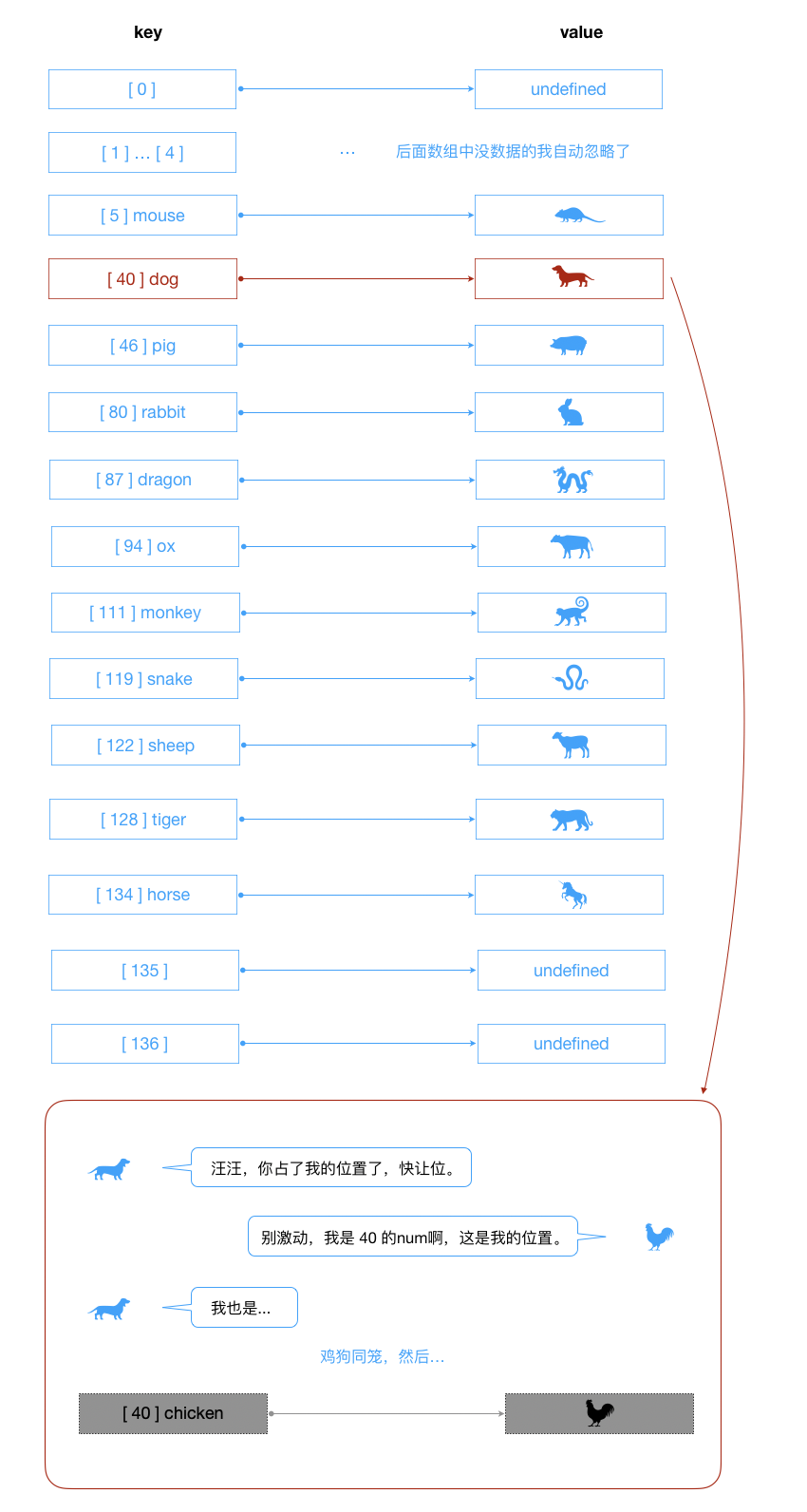1. 线性探测法

2. 开链法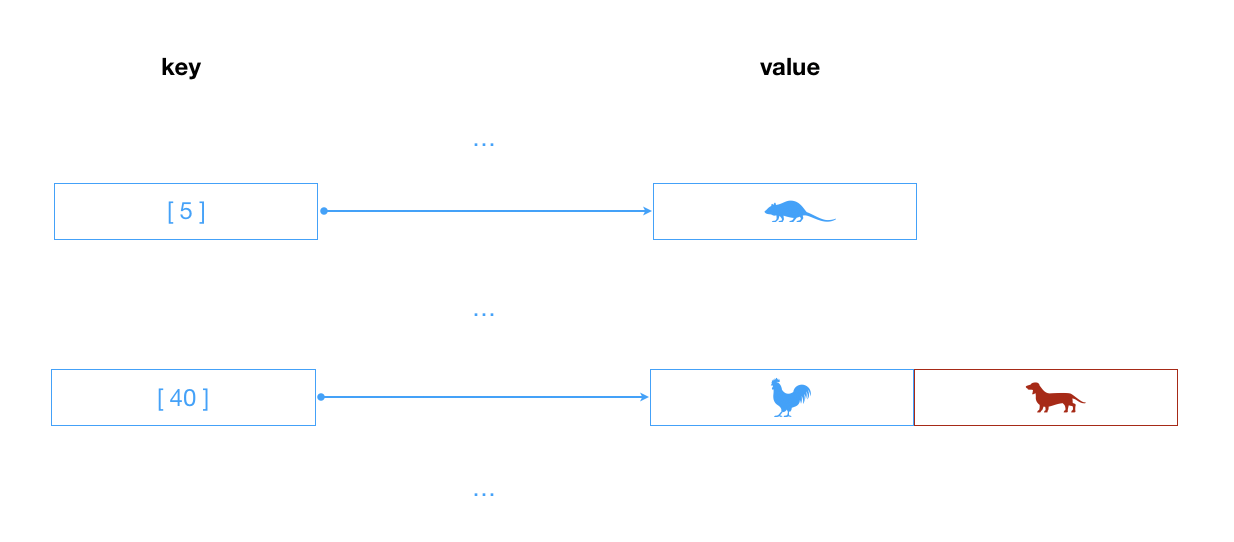### 二叉查找树

• 树的定义：

• 树（Tree）：`n(n >= 0)`个节点构成的有限集合。

• `n = 0`时，称为空树；
• 对任意一棵空树`(n > 0)`，它具备以下性质：
• 树中有一个称为根(Root)的特殊节点，用`r(root)`表示；
• 其余节点可分为`m(m > 0)`个互不相交的有限集`T1,T2,...Tm`，其中每个集合本省又是一棵树，称为原来树的子树（SubTree）
• 注意：

• 子树之间`不可以相交`
• 除了根节点外，每个节点有且仅有一个父节点；
• 一个`N`个节点的树有`N-1`条边。
• 树的术语：

• 节点的度（Degree）：节点的子树个数。
• 树的度：树的所有节点中最大的度数（树的度通常为节点个数的`N-1`）。
• 叶节点（Leaf）：度为`0`的节点（也称叶子节点）。
• 父节点（Parent）：有子树的节点是其子树的父节点。
• 子节点（Child）：若`A`节点是`B`节点的父节点，则称`B`节点是`A`节点的子节点。
• 兄弟节点（Sibling）：具有同一个父节点的各节点彼此是兄弟节点。
• 路径和路径长度：从节点`n1``nk`的路径为一个节点序列`n1,n2,n3,...,nk``ni``ni+1`的父节点。路径所包含边的个数为路径长度。
• 节点的层次（Level）：规定根节点在`第0层`，它的子节点是`第1层`，子节点的子节点是`第2层`，以此类推。
• 树的深度（Depth）：树中所有节点中的最大层次是这棵树的深度（因为上面是从第0层开始，深度 = 第最大层数 + 1）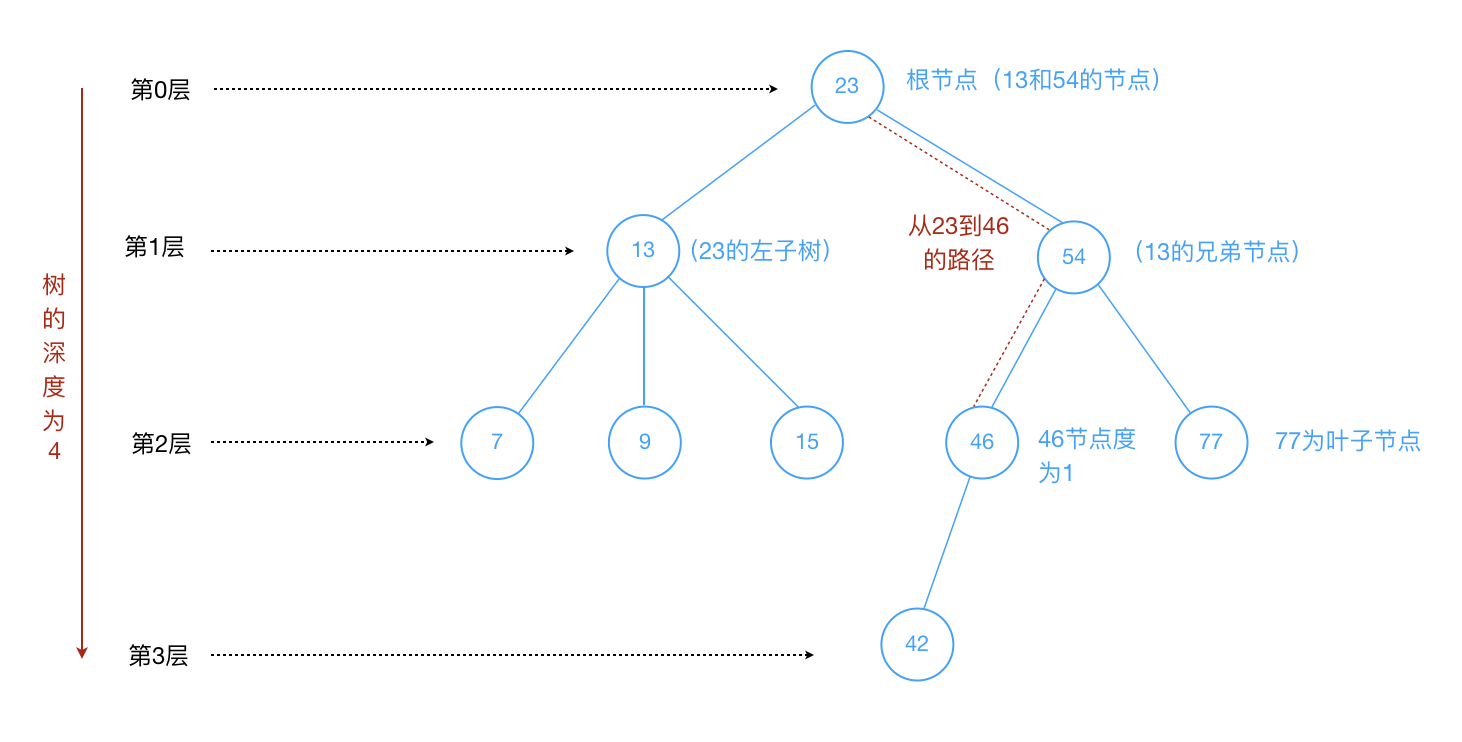• 二叉树的定义：

• 二叉树可以为空，也就是没有节点
• 二叉树若不为空，则它是由根节点和称为其左子树`TL`和右子树`RT`的两个不相交的二叉树组成
• 二叉树每个节点的子节点不允许超过`两个`
• 二叉树的五种形态：

• 只有根节点
• 只有左子树
• 只有右子树
• 左右子树均有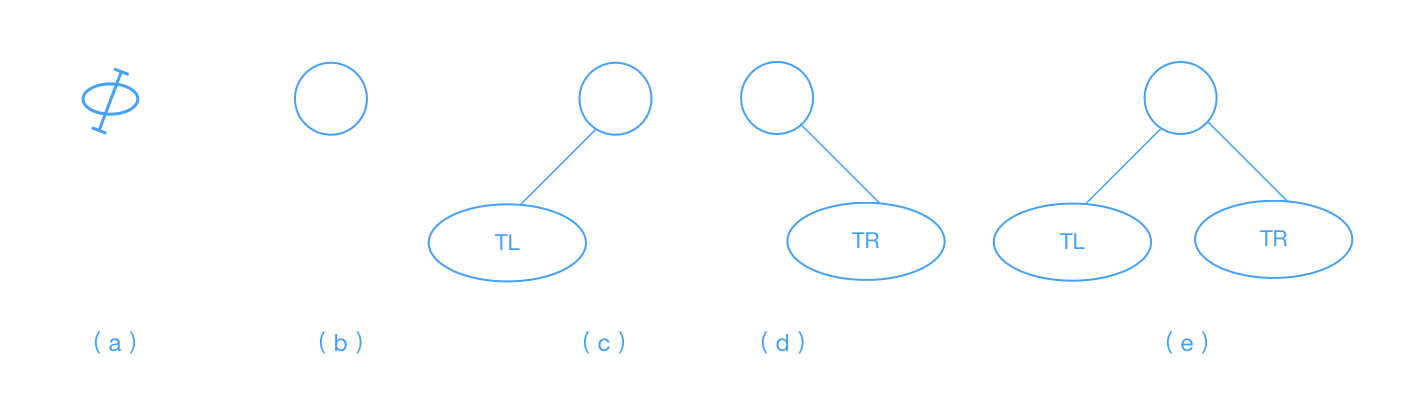``````javascript
// 二叉查找树
// 辅助节点类
class Node {
constructor(data, left, right){
this.data = data;
this.left = left;
this.right = right;
}
// 展示节点信息
show(){
return this.data;
}
}
class BST {
constructor(){
this.root = null;
}
// 插入数据
insert(data){
let n = new Node(data, null, null);
if(this.root == null){
this.root = n;
}else{
let current = this.root,
parent = null;
while(true){
parent = current;
if(data < current.data){
current = current.left;
if(current == null){
parent.left = n;
break;
}
}else{
current = current.right;
if(current == null){
parent.right = n;
break;
}
}
}
}
return this;
}
// 中序遍历
inOrder(node){
if(!(node == null)){
this.inOrder(node.left);
console.log(node.show());
this.inOrder(node.right);
}
}
//   先序遍历
preOrder(node){
if(!(node == null)){
console.log(node.show());
this.preOrder(node.left);
this.preOrder(node.right);
}
}
// 后序遍历
postOrder(node){
if(!(node == null)){
this.postOrder(node.left);
this.postOrder(node.right);
console.log(node.show());
}
}
// 获取最小值
getMin(){
let current = this.root;
while(!(current.left == null)){
current = current.left;
}
return current.data;
}
// 获取最大值
getMax(){
let current = this.root;
while(!(current.right == null)){
current = current.right;
}
return current.data;
}
// 查找给定的值
find(data){
let current = this.root;
while(current != null){
if(current.data == data){
return current;
}else if(data < current.data){
current = current.left;
}else{
current = current.right;
}
}
return null;
}
// 移除给定的值
remove(data){
root = this.removeNode(this.root, data);
return this;
}
// 移除给定值的辅助函数
removeNode(node, data){
if(node == null){
return null;
}
if(data == node.data){
// 叶子节点
if(node.left == null && node.right == null){
return null; // 此节点置空
}
// 没有左子树
if(node.left == null){
return node.right;
}
// 没有右子树
if(node.right == null){
return node.left;
}
// 有两个子节点的情况
let tempNode = this.getSmallest(node.right); // 获取右子树
node.data = tempNode.data; // 将其右子树的最小值赋值给删除的那个节点值
node.right = this.removeNode(node.right, tempNode.data); // 删除指定节点的下的最小值，也就是置其为空
return node;
}else if(data < node.data){
node.left = this.removeNode(node.left, data);
return node;
}else{
node.right = this.removeNode(node.right, data);
return node;
}
}
// 获取给定节点下的二叉树最小值的辅助函数
getSmallest(node){
if(node.left == null){
return node;
}else{
return this.getSmallest(node.left);
}
}
}

let bst = new BST();
bst.insert(56).insert(22).insert(10).insert(30).insert(81).insert(77).insert(92);
bst.inOrder(bst.root); // 10, 22, 30, 56, 77, 81, 92
console.log('--中序和先序遍历分割线--');
bst.preOrder(bst.root); // 56, 22, 10, 30, 81, 77, 92
console.log('--先序和后序遍历分割线--');
bst.postOrder(bst.root); // 10, 30, 22, 77, 92, 81, 56
console.log('--后序遍历和获取最小值分割线--');
console.log(bst.getMin()); // 10
console.log(bst.getMax()); // 92
console.log(bst.find(22)); // Node { data: 22, left: Node { data: 10, left: null, right: null }, right: Node { data: 30, left: null, right: null } }
// 我们删除节点值为22，然后用先序的方法遍历，如下
console.log('--移除22的分割线--')
console.log(bst.remove(22).inOrder(bst.root)); // 10, 30, 56, 77, 81, 92
``````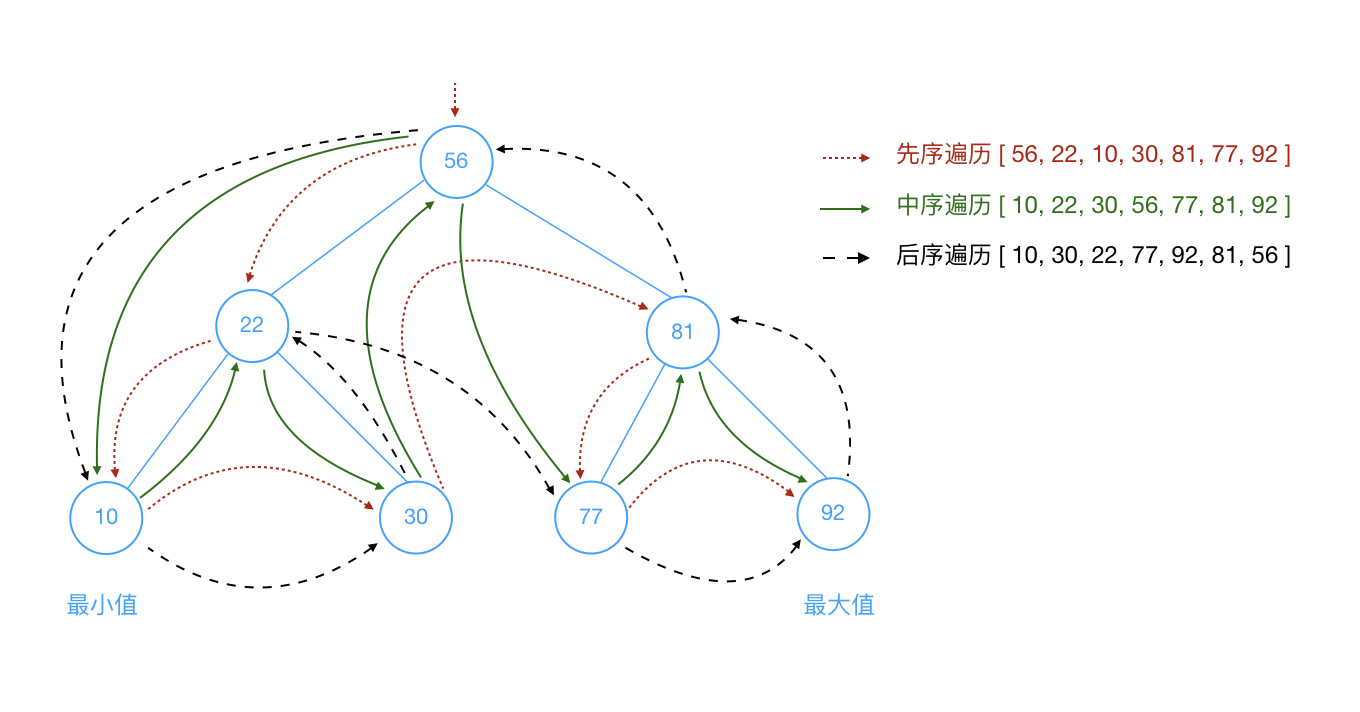• 该节点没有叶子节点的时候，直接将该节点置空；
• 该节点只有左子树，直接将该节点赋予左子树
• 该节点只有右子树，直接将该节点赋予右子树
• 该节点左右子树都有，有两种方法可以处理
• 方案一：从待删除节点的`左`子树找节点值`最大`的节点A，替换待删除节点值，并删除节点A
• 方案二：从待删除节点的`右`子树找节点值`最小`的节点A，替换待删除节点值，并删除节点A【👆上面的示例代码中就是这种方案】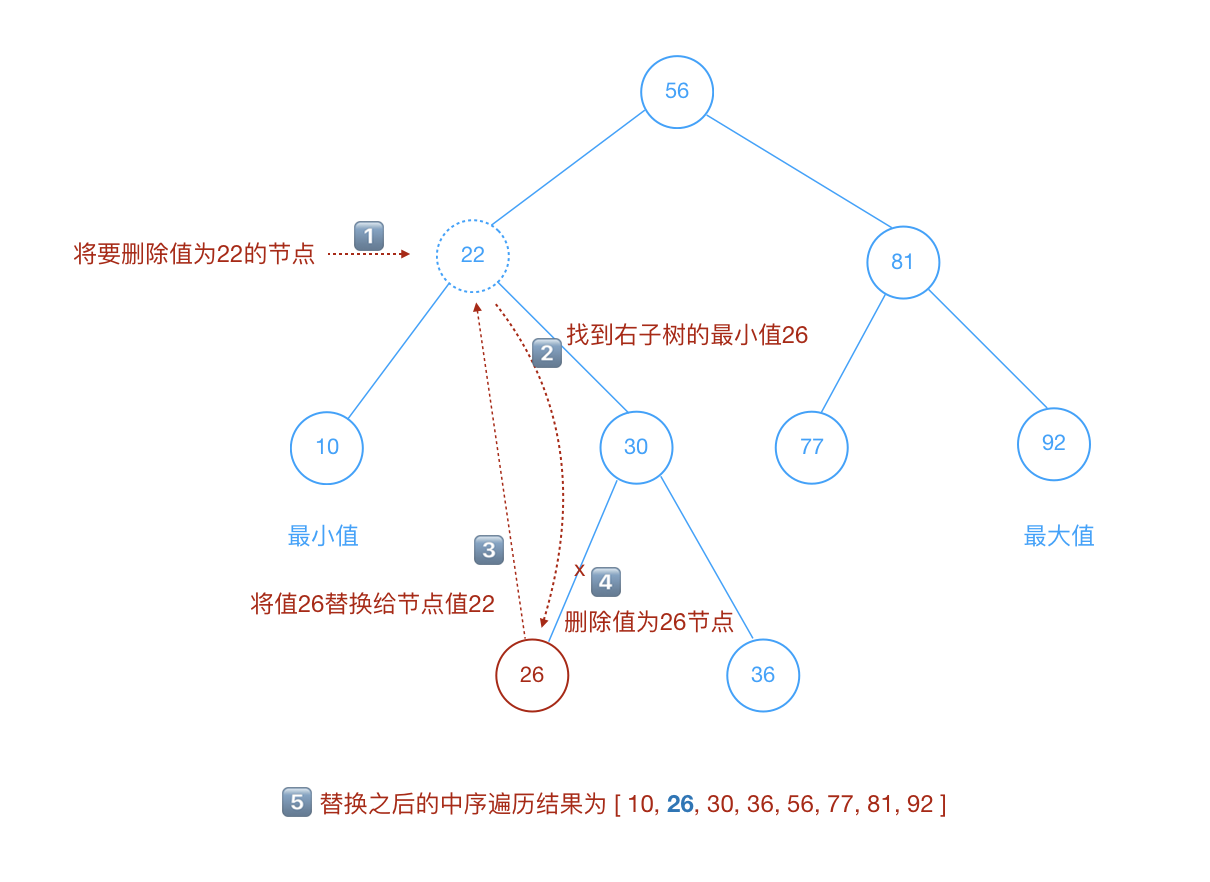### 图

• 顶点：图中的一个节点。
• 边：表示顶点和顶点之间的连线。
• 相邻顶点：由一条边连接在一起的顶点称为相邻顶点。
• 度：一个顶点的度是相邻顶点的数量。比如`0`顶点和其它两个顶点相连，`0`顶点的度就是`2`
• 路径：路径是顶点`v1,v2...,vn`的一个连续序列。
• 简单路径：简单路径要求不包含重复的顶点。
• 回路：第一个顶点和最后一个顶点相同的路径称为回路。
• 有向图和无向图
• 有向图表示图中的`边``有`方向的。
• 无向图表示图中的`边``无`方向的。
• 带权图和无权图
• 带权图表示图中的边`有权重`
• 无权图表示图中的边`无权重`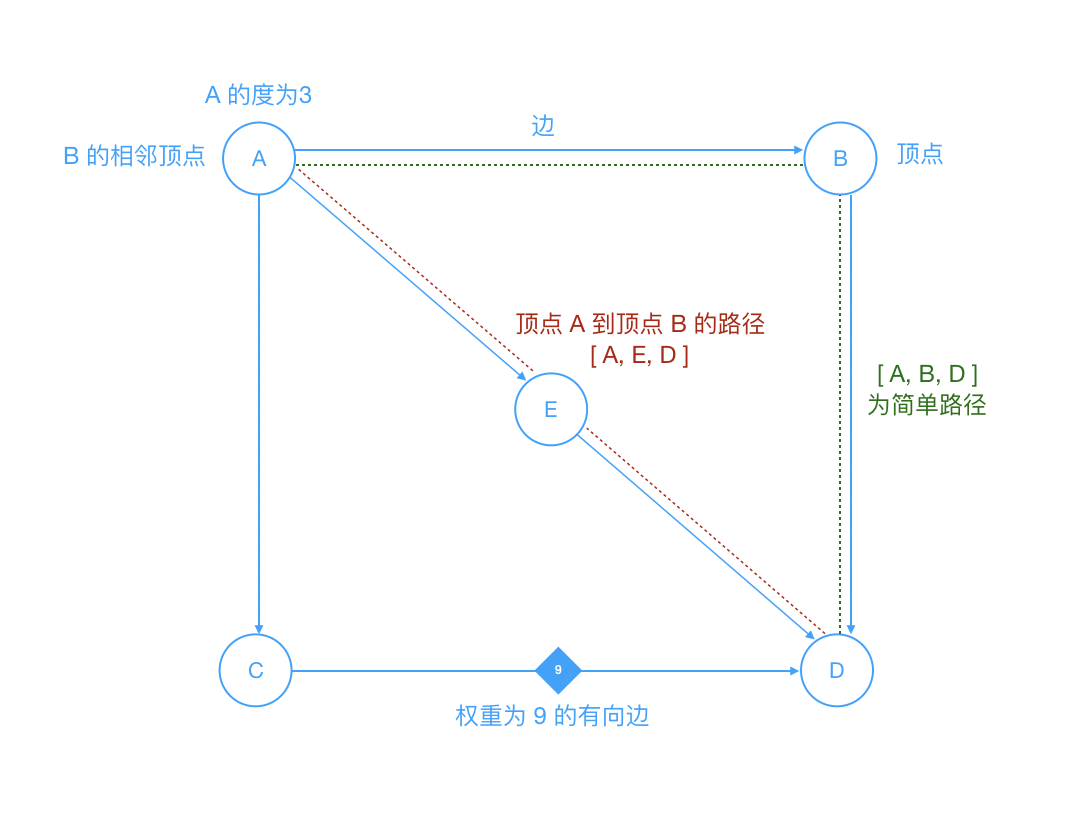• 对交通流量建模
• 顶点可以表示街道的十字路口, 边可以表示街道.
• 加权的边可以表示限速或者车道的数量或者街道的距离.
• 建模人员可以用这个系统来判定最佳路线以及最可能堵车的街道.

``````javascript
// 图
class Graph{
constructor(v){
this.vertices = v; // 顶点个数
this.edges = 0; // 边的个数
this.adj = []; // 邻接表或邻接表数组
this.marked = []; // 存储顶点是否被访问过的标识
this.init();
}
init(){
for(let i = 0; i < this.vertices; i++){
this.adj[i] = [];
this.marked[i] = false;
}
}
// 添加边
addEdge(v, w){
this.adj[v].push(w);
this.adj[w].push(v);
this.edges++;
return this;
}
// 展示图
showGraph(){
for(let i = 0; i < this.vertices; i++){
for(let j = 0; j < this.vertices; j++){
if(this.adj[i][j] != undefined){
console.log(i +' => ' + this.adj[i][j]);
}
}
}
}
// 深度优先搜索
dfs(v){
this.marked[v] = true;
if(this.adj[v] != undefined){
console.log("visited vertex: " + v);
}
this.adj[v].forEach(w => {
if(!this.marked[w]){
this.dfs(w);
}
})
}
// 广度优先搜索
bfs(v){
let queue = [];
this.marked[v] = true;
queue.push(v); // 添加到队尾
while(queue.length > 0){
let v = queue.shift(); // 从对首移除
if(v != undefined){
console.log("visited vertex: " + v);
}
this.adj[v].forEach(w => {
if(!this.marked[w]){
this.marked[w] = true;
queue.push(w);
}
})
}
}
}

let graphFirstInstance = new Graph(5);
graphFirstInstance.addEdge(0, 1).addEdge(0, 2).addEdge(1, 3).addEdge(2, 4);
graphFirstInstance.showGraph();
// 0 => 1
// 0 => 2
// 1 => 0
// 1 => 3
// 2 => 0
// 2 => 4
// 3 => 1
// 4 => 2
// ❓为什么会出现这种数据呢？它对应的图是什么呢？可以思考🤔下，动手画画图什么的
console.log('--展示图和深度优先搜索的分隔线--');
graphFirstInstance.dfs(0); // 从顶点 0 开始的深度搜索
// visited vertex: 0
// visited vertex: 1
// visited vertex: 3
// visited vertex: 2
// visited vertex: 4
console.log('--深度优先搜索和广度优先搜索的分隔线--');
let graphSecondInstance = new Graph(5);
graphSecondInstance.addEdge(0, 1).addEdge(0, 2).addEdge(1, 3).addEdge(2, 4);
graphSecondInstance.bfs(0); // 从顶点 0 开始的广度搜索
// visited vertex: 0
// visited vertex: 1
// visited vertex: 2
// visited vertex: 3
// visited vertex: 4
``````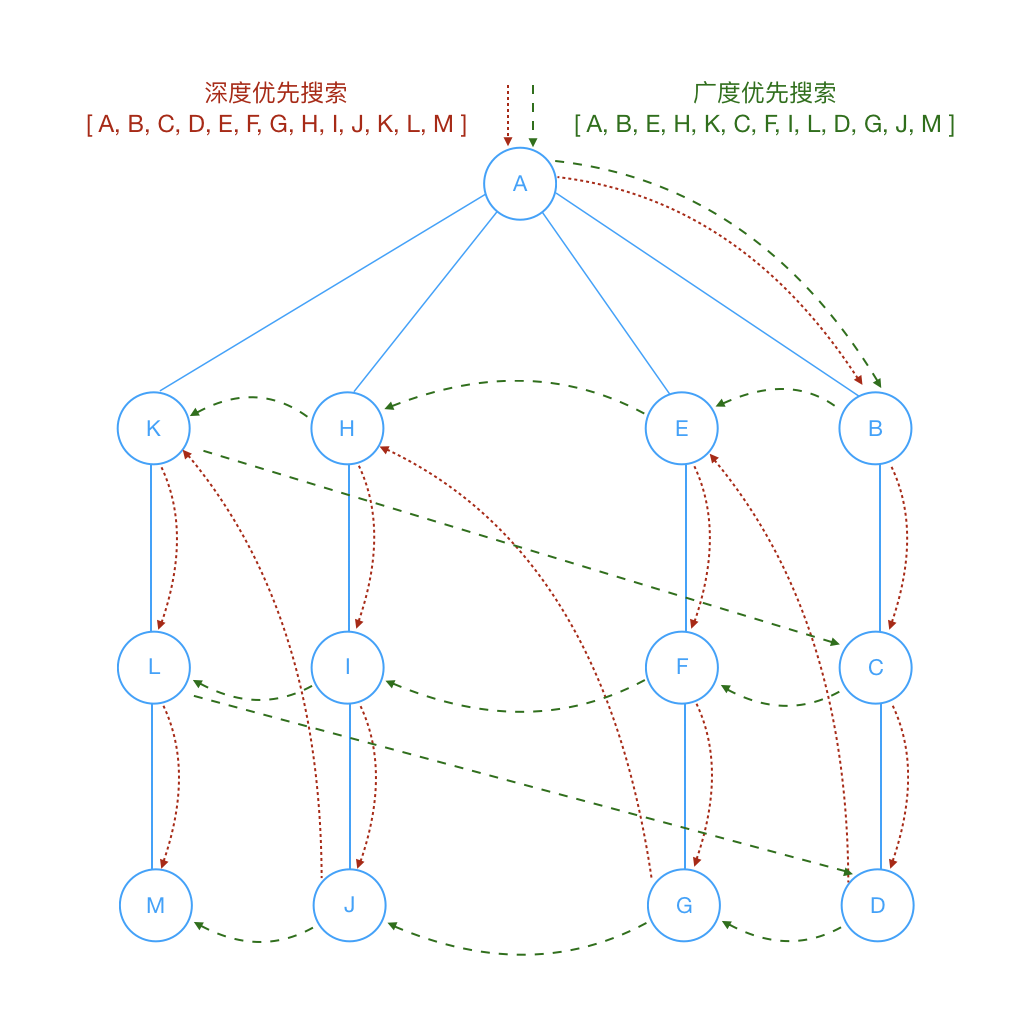### 后话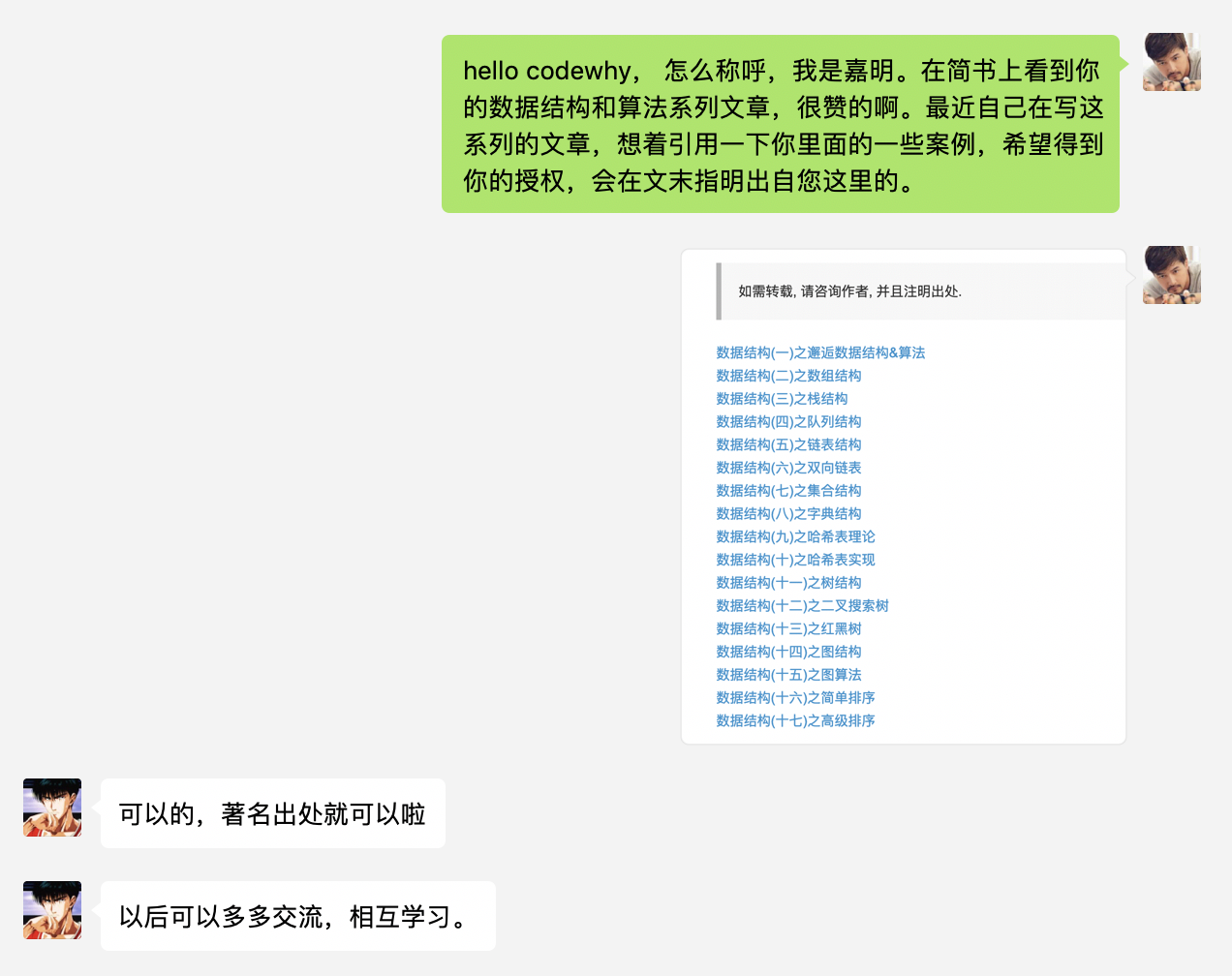### 参考

• 点赞
• 写回答
• 关注问题
• 收藏
• 复制链接分享
• 邀请回答

#### 6条回答

• 很棒！看了一遍打算star，结果发现已经star过了

点赞 评论 复制链接分享
• Thank you!

点赞 评论 复制链接分享
• 您好，我想转载到我自己的GitHub上，翻译成英文版本，会直接注明翻译自您的文章，图片打算直接用您的，汉语的部分用英文翻译遮住。 请问是否可以？

点赞 评论 复制链接分享
• 👌，著名出处就行了

点赞 评论 复制链接分享
• link-list的移出元素需要让length--

点赞 评论 复制链接分享
• -96 谢谢提醒，已加上`this.length--`

点赞 评论 复制链接分享